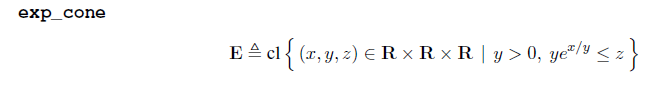# What is the difference between `exponential` and `exp_cone`?

In many answers, e.g., How to express “x*(2^(1/x)-1)” in CVX
, `exponential(1)` is used.

What is the difference between `exponential` and `exp_cone`?

I have tried to find `exponential` in CVX manual, but I cannot find it.

There are only `exp_cone`, defined asI think to minimize x*(2^{1/x}-1), I have to use the right-most code as follows.

However, in How to express “x*(2^(1/x)-1)” in CVX
, Mark_L_Stone recommended to use the following code: `{1,x,z} == exponential(1)`

Please let me know what the difference is between `exponential` and `exp_cone`?
Also, why he recommended to use `==` instead of `<in>`?

Thank you.

The CVX Users’ Guide erroneously shows `exp_cone`. The actual implemented syntax uses `exponential`, as used in my answers.

I don’t believe
`<in> exp_cone`
works, because CVX does not recognize `<in>`, at last when I just tried it in CVX 2.2

1 Like

Here, does `==` imply “in”? That is, is the phrase `{1,x,z} == exponential(1)` representing that a tuple (1,x,z) is in a set exp_cone?

The syntax `{1,x,z} == exponential(1)` works. i don’t know whether any other syntax works.

I didn’t design or develop CVX. I’m just telling you what works. I suggest you use that.

1 Like